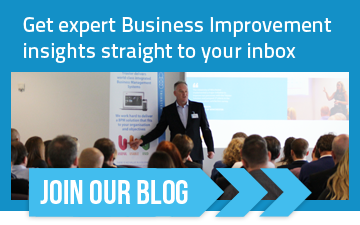# Process Costing: A Simple Method to Find How Much Your Processes Cost

In virtually every organization one of the key aims is to save your business money through process costing and the identification of more efficient processes. Cost is one example of a quantitative metric and the advice outlined in this article applies equally to any quantitative metric, for example one could just as easily focus on amount of effort involved, numbers of people, elapsed time from start to finish, the amount of defects introduced, the amount of customer complaints received and so on...What we really need is a simple method for working out how much this process is costing us, and to then compare it with the alternatives."

To work with quantitative metrics is straightforward, but it has to be rigorous. In this article I'll cover the ground on some of the underlying theory and definitions, and also look at what is process costing with examples. First though, we need to understand several concepts:

1. The Noun-Verb method and how it relates to metrics
2. Atomic and non-atomic data
3. End-to-end processes
4. The process hierarchy seabed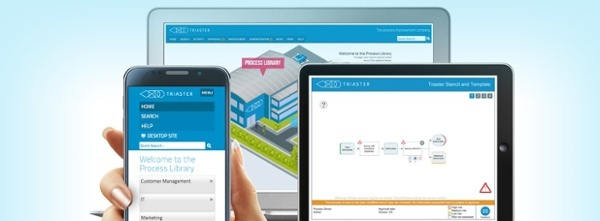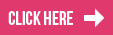## Quantitative Metrics and the Noun Verb method

In the Noun-Verb process mapping method, Activities are described using a verb, and Deliverables (the outcome of performing an Activity) are described using Nouns.

When working out process costs in an attempt to save your company money, the costs should always be exclusively associated with the Activities. Activities represent work performed, they genuinely reflect where costs are incurred in a process. Deliverables on the other hand represent the benefit of performing work, they are in some sense the opposite of a cost, they are the return on investment of the cost of the producing Activity.

As shall be explained in the next section, cost need not be a fixed value, it can be a formula referencing other more basic information. This is good practice wherever possible.Only attach cost values to Activities (or Decisions), never to Deliverables.

## Atomic and non-Atomic Data

Atomic Data are values that cannot be broken down or derived from other atomic values (hence atomic or atom-like meaning indivisible. As an aside this is an unfortunate historical use of the term from the days when it was believed an atom could not be sub-divided - how wrong we were!)

My age for example might appear to be atomic, but on closer inspection my age can be derived from my date of birth. My date of birth is therefore atomic, my age is non-atomic. My weight on the other hand is atomic - it is not possible to work out my weight from any other values.

When dealing with process costs, it is important to understand when to simply attach costs to a process as if cost were an atomic value, and when to derive the cost from other data. More often than not, it is desirable to derive cost.

For example, suppose I am trying to calculate the labour cost of a process that involves 3 people working for 6 hours at a rate of £8 per hour, and a supervisor's time of 1 hour at a rate of £16 per hour. The atomic data in this example are the number of hours worked, and the rate per hour. The cost can be derived from these values and so therefore cost should not be recorded as an atomic data value in this process, but as a derived value from other data.

On the other hand, suppose I am trying to work out the labour cost of a process where the number of hours worked is not known, nor the rate per hour, but a contract is in place with an outsourced business to perform the process at a cost of £800 per day. Then in this instance cost is an atomic data value and it should therefore be recorded as a data value in the process.Wherever possible, use atomic data and then derive costs rather than enter a fixed cost.

## End-to End Process Costing

Anyone familiar with a Process Library will know it is possible to view a process as a sequence of Activities beginning with an Input and ending with an Output. The end-to-end process cost is simply the average cost of performing the end-to-end process many times over. Sounds simple? But please note use of the word average in that sentence.

It is necessary to consider the concept of an average because processes sometimes have branch points in them. On one execution of the process the branch will go one way, and on another it will go the other way. Sometimes there are exceptional circumstances that require an exception process to be performed. Not only are there branch points, sometimes there are loopbacks, and on a single run of a process, the same process element can be executed many times over, each of which adds to actual cost.

An end-to-end process cost therefore isn't simply the sum of the cost of each Activity in the process, it is defined more precisely as:The end-to-end process cost is the sum of the cost of each process element multiplied by the expected number of times that process element is executed. Expected number of times can be any number from 0 upwards, and fractions are allowed.

Let's take a few simple examples.

We shall start with a simple end-to-end process with no branches or loopbacks. An example can be found here in the Execute Project Analysis process.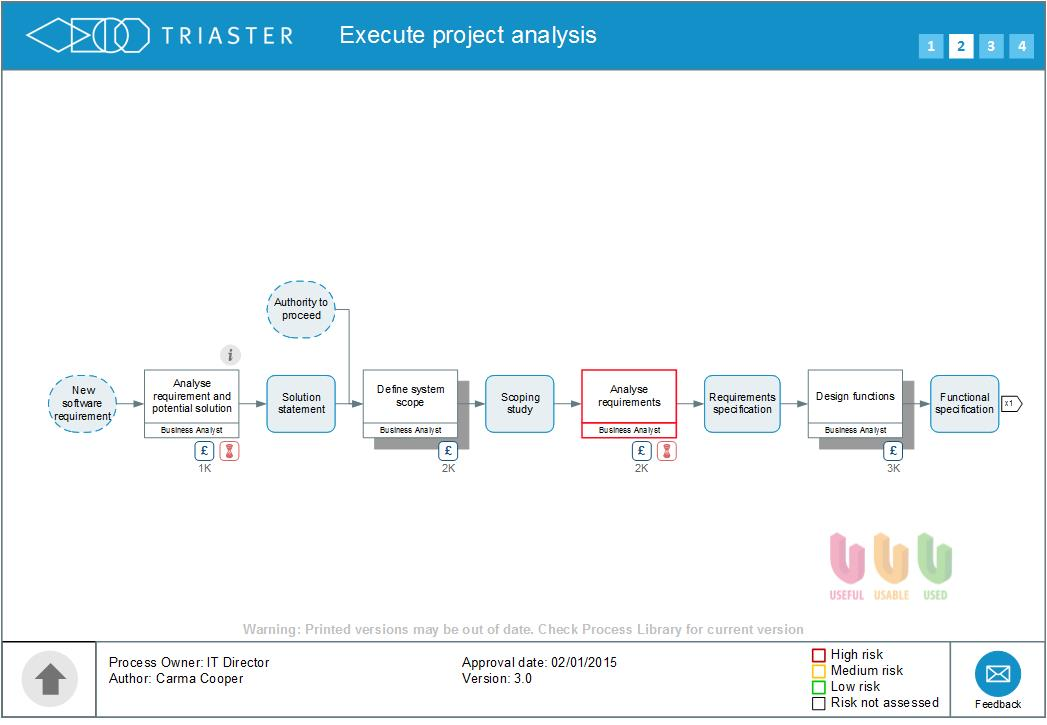Because each execution of the process must use every Activity, and does so only once, then the end-to-end process cost is simply the sum of the four Activity costs (in the interactive version control-click to see the cost of each Activity).

At the time of writing, that gives an end-to-end process cost of £72,850. In the interactive version control-click on the text "Execute project analysis" to see the Aggregate Cost of the whole process.

As a second example, please refer to the IT Life Cycle process shown below: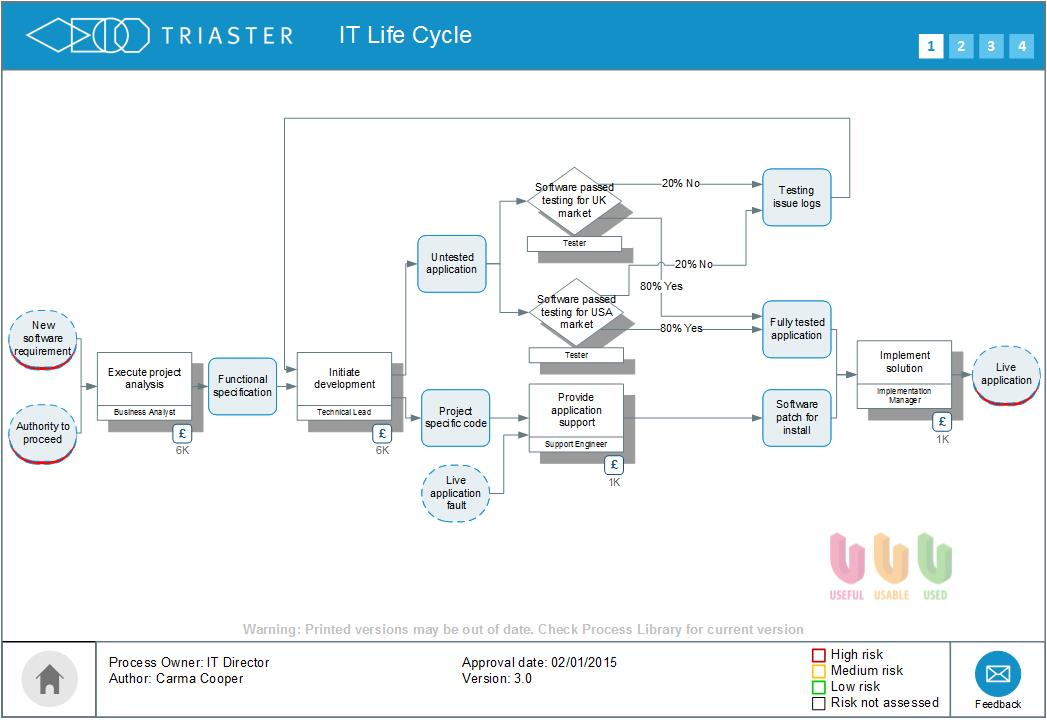The end-to-end process branch contains 2 decision points, both of which are also loopbacks. So, in this process, we need to take into account the branch likelihood when calculating the cost because re-work will happen from time to time.

In the case of a loop back the relationship between the probability of a branch being executed, and the number of times the loop executes (N) is given by:N = p/(1-p)

where p is the branch probability. So, in the IT Life Cycle, the loopback occurs 20% of the time, N is therefore 0.2/0.8 = 0.25, i.e. the loop occurs on average 1 in 4 instances of the process. If the probability were say 95%, then N is 0.95/0.05 = 19, i.e. the loop executes on average 19 times.

The cost of the end-to-end process therefore should contain an additional N * loop cost whenever there is a loopback.

In the example, the repeated step is Initiate Development which costs £121,700. A quarter of this is £30,425, but as both decisions loop to it, the contribution to cost is taken from both loops and we therefore double the contribution to £60,850. The total cost of this process is therefore the total cost of the Activities in the process plus a further £60,850 - a grand total in this instance of £278,025.

## Process Hierarchy and Process Seabed Costs

It will be understood that end-to-end is the sense of running from left to right from a set of Inputs to a set of Outputs. Any end-to-end process can therefore be modeled as a single Activity with the initial set of Inputs and final set of Outputs - even if the Activity itself is representing tens or even hundreds of more detailed Activities found in the end-to-end process.

This grouping is generally modeled across several vertical levels so that on any given level the Activities represent a sensible grouping of Activities on the level beneath - one can then 'drill-down' and 'drill-up' between higher level summaries and their lower level more detailed expansions.

The cost of an Activity that is itself a summary of Activities on more detailed levels is therefore non-atomic and is always derived and must be equal to the cost of the end-to-end process it summarises or drills-down to. Of course, any given level in a process hierarchy itself also can be considered as a set of end-to-end processes, so in the computation of costs at a higher level, one simply applies the rules outlined in the previous section regarding how to compute the end-to-end process costing.Only enter cost information into the seabed layer of the process, i.e. on Activities that have no drill-down. For every map, store the Aggregate Cost in the Node of the Map, this can then be used automatically as the Activity cost on higher levels.

## Automation and Data Managers

Most business process modelling tools will have the ability to automate all of these considerations to some degree or other. With the Triaster solution, to ensure complete customer control over costing and ease of use, we have produced Excel-based Data Manager files that provide automated computation of costs (end-to-end and hierarchical) and the ability to fine tune the calculations.

## Conclusion and Next Steps

The technique described in this article shows how robust process costs can be derived from entering atomic cost information into the seabed of a process. Once the base values are entered, all other data can be computed automatically so that an end-to-end or hierarchy cost emerges from the model.

A simple formula has been given to show how loopbacks affect the cost of the end-to-end process.

The same principles can apply to any quantitative metric - cost is just an example that is most commonly required by Triaster customers.Don't forget to leave a comment as we are always interested in receiving your insights and share the article using our social buttons if you found it useful and you think that others might feel the same.

## Related Articles:

How to Cost a Business Process Management (BPM) System

How much does a Business Process Management (BPM) software system cost?

Problems with Business Process Management (BPM): Getting employees to follow the process

7 Key Questions to ask when shopping for Business Process Management software### Join over 3,000 Quality and Improvement professionals

Get expert Process Mapping, Management and Improvement insights straight into your inbox.

### Posts by Topic